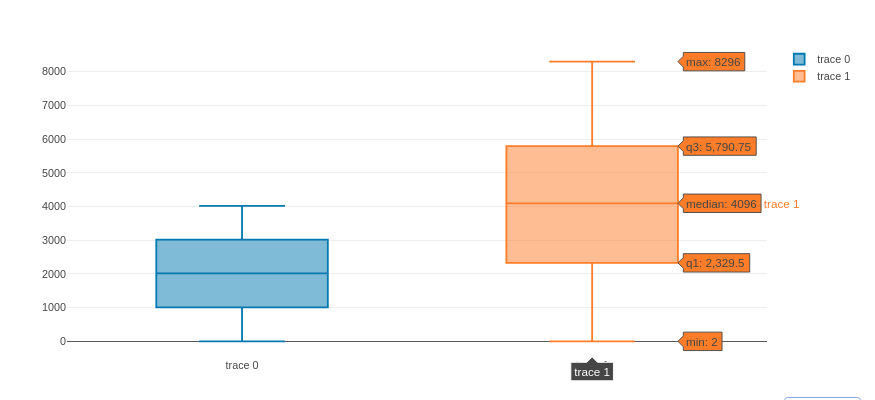• #5, First Floor, 4th Street Dr. Subbarayan Nagar Kodambakkam, Chennai-600 024 Landmark : Samiyar Madam
• pro@slogix.in
• +91- 81240 01111

### How to detect outliers using plotly in python?

###### Description

To create box plot to detect the outliers in a data set variable.

###### Process

Scaling the feature variables.

Initialize the box plot object form plotly library.

Declare the plotting variables.

Pass it into the go.box(variable).

Use py.iplot(variable) to create the box plot.

Box plot used to detect the outliers attributes in a variable.

###### Sample Code

#import plotly library

import plotly.plotly as py

import plotly.graph_objs as go

#import pandas library

import pandas as pd

Sathish/Pythonfiles/flyer.xlsx’)

df=pd.DataFrame(data)

#declare the variable

y0 = df[‘FlyingReturnsMiles’]

trace0 = go.Box(y=y0)

#store it in a variable

#because plotting object takes 1 argument only

data1 = [trace0]

#plotting the variable

py.iplot(data1)

Source code(Two variable):

#import plotly library

import plotly.plotly as py

import plotly.graph_objs as go

#import pandas library

import pandas as pd

Sathish/Pythonfiles/flyer.xlsx’)

df=pd.DataFrame(data)

#declare the variable

y0 = df[‘FFP#’]

y1 = df[‘EnrollDuration’]

trace0 = go.Box(y=y0)

trace1 = go.Box(y=y1)

#store it in a variable

#because plotting object takes 1 argument only

data1 = [trace0,trace1]

py.iplot(data1)

###### Screenshots